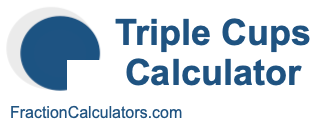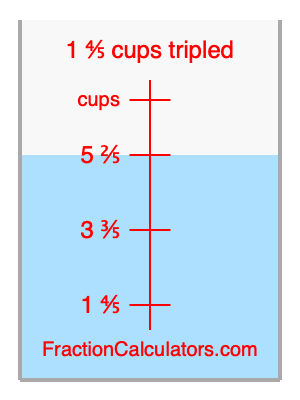What is 1 4/5 cups tripled?Here we will calculate 1 4/5 cups tripled and illustrate 1 4/5 cups tripled on a measuring cup. What is 1 4/5 cups tripled? 1 4/5 cups tripled is 1 4/5 cups times three. Therefore, to triple 1 4/5 cups, we multiply 1 4/5 by 3. Here is the formula, the math, and the answer to 1 4/5 cups tripled:

Cup × 3 = Cup tripled
1 4/5 × 3 = 5 2/5
1 4/5 cups tripled = 5 2/5 cups

Below is an illustration of a measuring cup. We show 1 4/5 cups and 1 4/5 cups tripled (5 2/5 cups) so you can see where they are in relation to each other.As you can see, we filled up our measuring cup to 1 4/5 cups tripled. In other words, we filled it up to 5 2/5, which is three times 1 4/5.

Triple Cups Calculator
Here you can triple another cup measurement.

What is cups tripled?

What is 1 5/6 cups tripled?
Here is the next measurement on our list that we have tripled for you.